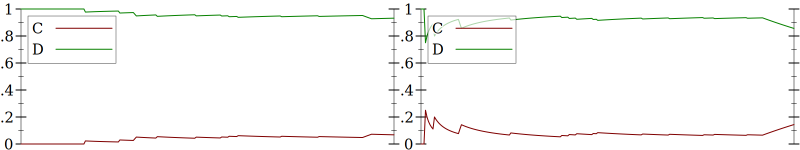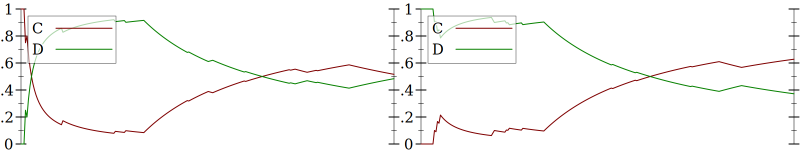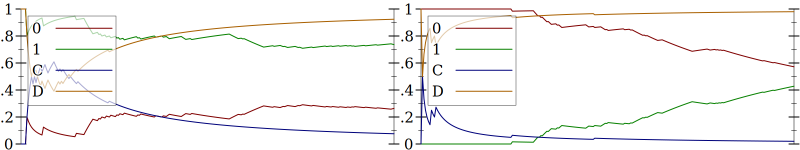## By Pavel Panchekha

### 29 December 2018

Share under CC-BY-SA.

# Learning Shame

In a previous post, I discussed a twist on the Prisoner's Dilemma, wherein it is rational for agents to “feel shame”: to modify their utility function to decrease the utility of defecting against cooperators. What's neat is that it is a fully rational micro-foundation for getting cooperation in the PD.

Today, I want to look whether this behavior can be learned.

## Learning to play

One line of research in game theory is in determining how game-theoretic agents learn to play the game they are in effectively. Finding Nash equilibria is NP-hard (ish1 [1 It's PPAD-complete; NP doesn't apply because there always is a solution, it's just hard to say what it is.]), so most work looks either at looser notions of equilibria2 [2 If there are more equilibria, then they're easier to find.] or at subsets of games.3 [3 Van Neuman's theorem gives a P-time algorithm for solving two-player zero-sum games.] I picked up N. Cesa-Bianchi and G. Lugosi's “Prediction, Learning, and Games” to learn a bit about this field, and ended up choosing two algorithms to learn shame.

Generally speaking, learning algorithms differ not only on the sort of equilibrium they guarantee but also on the information they use. Some algorithms are blind: they only use the moves taken and rewards earned in normal game-play to learn. Other algorithms additionally use counterfactual information: what would the reward have been if a different strategy were used? In the "learning shame" experiment there are two decisions to make: first whether to feel shame, second what to do. I decided to use a blind algorithm for the first choice (since it's hard to make counterfactual decisions about the results from different utility functions) and a regular algorithm for the second (since at that point we are playing a normal game).

## No-regret learning algorithms

Both algorithms are pretty simple.

To learn using the which move to make, given a utility function, we store a vector of weights, one per action. Those weights are the sum of the utilities we would have earned, at each step, from taking that action. Then the actual action to take is chosen from the soft-max distribution on the weights. So, for example, suppose we are playing the normal prisoner's dilemma, with outcomes:

 C D C 5, 5 0, 6 D 6, 0 1, 1

Also suppose that we play three times: C/C, D/C, C/D. Then for the first player, the weights would have been:

 Turn 1 Turn 2 Turn 3 C 5 5 0 D 6 6 1

The weights are now 10 for C and 13 for D, so we will choose to cooperate with probability $p_C = \frac{\exp(10t)}{\exp(10t) + \exp(13t)}$ where $$t$$ is a temperature parameter. For example, if $$t = 0.1$$, as it is in my simulation, this gives a probability of 42% for cooperating.

Since defection always earns exactly 1 utility more than defecting, the probability of cooperation after $$n$$ turns will be $$1 / (1 + \exp(nt))$$ and we can see that in this case the algorithm correctly learns the optimal behavior. More generally, this algorithm always converges to players playing a coarse correlated equilibrium. That's a relatively weak kind of equilibrium, but in the PD there's only one of them, so of course this algorithm learns it.

The algorithm for learning without counterfactual information is pretty similar to the above, but you slowly dilute the weights. This compensates for the fact that you only have a reward amount for one action. This algorithm works more slowly than the previous algorithm, since it has less information to go off of.

Note that for numerical reasons it's best to normalize the weight vector so that the smallest weight is zero.

## Results

I implemented both algorithms in Racket and ran them both on the original PD and on the one where agents can choose to feel shame.

Both algorithms successfully learn to play the prisoner's dilemma. Here, for example, is a plot of the non-blind algorithm playing the prisoner's dilemma:Figure 1: Reproduce this plot with (plot-results pd-game (run-game pd-game))

In this plot, the C and D lines track the probability of playing cooperate or defect, and the horizontal axis counts the learning steps. Moreover, they not only learn the prisoner's dilemma where shame is mandatory, but they also learn to cooperate:Figure 2: Reproduce this plot with (plot-results pd2-game (run-game pd2-game))

Here, you can see that they initially learn to defect, but then moderate their approach. The modified prisoner's dilemma (where shame is mandatory) allows any mix of cooperate and defect as a coarse correlated equilibrium, so this outcome is possible—though, over time, the details of the algorithm cause it to converge on the all-cooperate equilibrium.

However, the combined "learning shame" experiment is mostly a failure:Figure 3: Reproduce this plot with (plot-results pd-game (run-moral-game pd-game pd-util+))

Here you can see that the agents learn not to cooperate, and since they're already not cooperating, they have no reason to choose one or the other utility function (so the probabilities of those move around at random).

## Why didn't it work?

The key reason for this—the key reason why the agents don't learn to be nice to one another—is that they do not know whether the other agent will feel shame or not. Since the only good move against a shameless agent is to defect, and since this is also a reasonable move against a shameable opponent, defect is the obvious choice (it is weakly dominant); and once you've chosen that, there's no difference whether or not you feeling shame.4 [4 Remember that feeling shame only means a utility hit to defecting on cooperators; since you expect your opponent to defect, it doesn't matter.]

So, for the sort of free choice to feel shame to work, there have to be ways to credibly signal your choice of utility function. There are a variety of ways this could happen. First, perhaps the choice to feel shame could be quite broad (feel shame in a large variety of different games); then your behavior in those games could credibly signal your change in utility function. Or, there could un-fakeable signals of shame (flush cheeks, metaphorically). However, both of these mechanisms are pretty heavy-weight. Overall, it seems that there is not a simple method to learn to feel shame.

## Footnotes:

1

It's PPAD-complete; NP doesn't apply because there always is a solution, it's just hard to say what it is.

2

If there are more equilibria, then they're easier to find.

3

Van Neuman's theorem gives a P-time algorithm for solving two-player zero-sum games.

4

Remember that feeling shame only means a utility hit to defecting on cooperators; since you expect your opponent to defect, it doesn't matter.